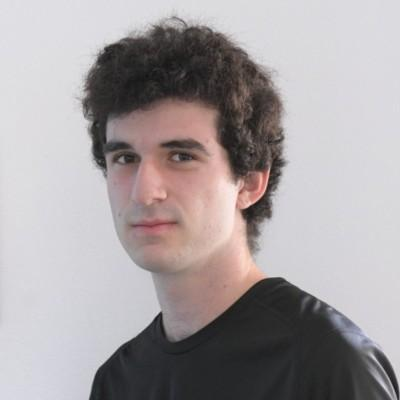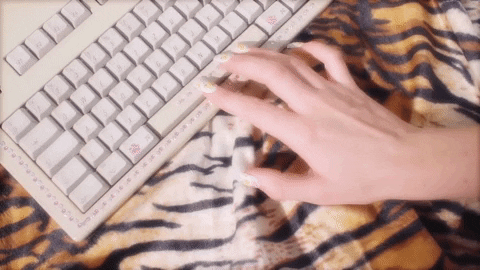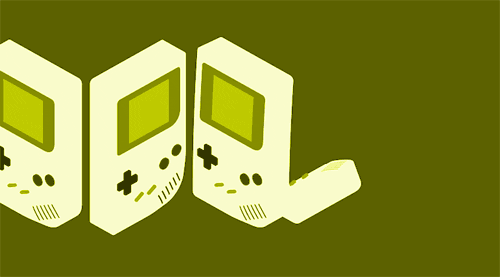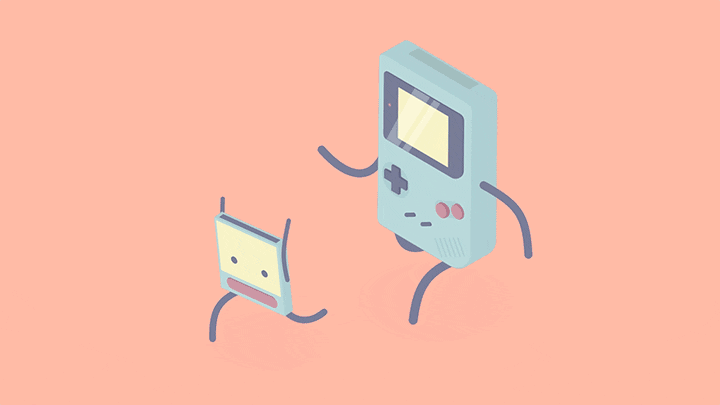# Space Bar Games

## Make two games where you press the space bar as fast as possible!@JakeGerber

We all love spamming the space bar so let's make a game based on it. In this workshop, we will be creating a two space bar games. In the first you have to press 100 times as fast as possible, and in the second you have to press has much as possible in 5 seconds.Here's a demo of what we're going to create!## Repl.it Setup

We're going to use Repl.it, a free, online coding editor, to create the project. Get started by visiting repl.it/languages/python3.## Importing Libraries

Let's begin by importing the turtle and math libraries. We need these to access certain functions!

``````import time
import turtle
``````

We will be drawing to the screen using the turtle library! Turtle allows us to draw to the screen like a whiteboard. We're going to use it to draw text to the screen. More information here. The time library will be used for time! We want this so we can track the time for both of the games.

## Initial StatementsInitial statements are needed. Don't forget them! Put them right under the import statements.
``````turtle.hideturtle()
turtle.up()
turtle.goto(-150,0)
``````

These initial turtle statements hide the cursor, make the turtle go up to prevent it from drawing when we change locations, and go to a specified location. This location is where we will be drawing some text.

This code goes at the bottom.

``````start = 0
spacePress = 0
turtle.write("Press Left for Space Game 1 Right for Space Game 2", font=("Arial", 8, "normal"))
``````
• The `start` integer is initialized. It will be used in Game 1 to get the user's starting time. This will be subtracted from the ending time to get the total time.
• The `spacePress` integer is initialized. It will be used in both games when the user presses the space bar.
• Finally, the turtle writes to the screen how to start the games.

## Initial Booleans

This code goes at the bottom.

``````game1Playing = False
game1end = False

game2Playing = False
game2end = False
timerActive = False
``````

These booleans are initialized. They will be used later on when the user starts playing a game.

## Left Arrow Press FunctionTo the left now.

Game 1 is the game where we will be pressing the space bar as fast as possible for 100 times. This function will be at the bottom of the program.

``````def left():
global game1Playing
global start
game1Playing = True
start = time.time()
``````
• This code will occur when the user presses the left arrow key to start Game 1, which we will handle later on.
• We are making some booleans global so we can access and modify them here. When the `global` keyword is used inside of a function, we are able to access the variable and modify it. Without this keyword, we cannot do anything with the variables we created earlier in our program.
• Set `start` to the current time. Remember, this will be used later on to get the total time of the user.

This code goes within the `left` function and right under what we just wrote.

``````def left():
#What we just wrote would be here.
turtle.clear()
turtle.goto(-100,0)
turtle.write("Click Space as Much as Possible", font=("Arial", 10, "normal"))
``````

The turtle clears the screen, goes to a specified location, and tells the user the directions for Game 1.

## Right Arrow Press FunctionTo the right now.

Game 2 is the game where we will be pressing the space bar as much as possible in 5 seconds. This function goes right under the `left` function.

``````def right():
global game2Playing
game2Playing = True
``````
• This code will occur when the user presses the right arrow key to start Game 2, which we will handle later on.
• Make the `game2Playing` boolean global so we can access and modify it. In fact, set it to true after we make it global. Without it being global, we would not be able to do this.

This code goes within the `right` function and right under what we just wrote.

``````def right():
#What we just wrote would be here.
turtle.clear()
turtle.goto(-100,0)
turtle.write("Click Space as Much as Possible", font=("Arial", 10, "normal"))
``````

The turtle clears the screen, goes to the specified location, and tells the user the directions for Game 2.

## Activate Timer

This code will be used for Game 2, and the function will be called later in the program. It will make more sense then so don't worry! This function does right below our `right` function.

``````def TimerActivate():
global game2end
timeVal = 5
``````
• This function will tell the user how much time they have left to press the space bar before the game ends.
• Make the `game2end` boolean global so we can access it.
• Create the `timeVal` integer and set it equal to 5. This represents the amount of the time the user has to press the space bar as fast as possible in Game 2.

This code goes within the `TimerActive` function and right below what we just wrote.

``````def TimerActivate():
#What we just wrote would be here.
for i in range(timeVal):
turtle.clear()
turtle.goto(-100,0)
turtle.write("Seconds Remaining: " + str(timeVal - i), font=("Arial", 10, "normal"))
time.sleep(1)
game2end = True
``````
• This for loop will run `timeVal` times. But how will it be `timeVal` seconds long? That's where the `time.sleep(1)` line comes in, which will wait one second before running the loop again. This creates our five second timer.
• Within the loop, clear then screen, go to the specified coordinate, and led the user know how much time they have remaining.
• After the loop, set the game2end boolean to true to represent the game's end.

## Space BarThe user's going to be pressing space so let's handle that. This is also where it will check what game is currently being played and have code for both games. This function goes at the bottom of the program.

### Initial Variables

``````def space():
global game1Playing
global spacePress
global timerActive
global game2Playing
spacePressAmt = 100
``````

Create a new function and add these global statements so we can use these booleans. The `spacePressAmt` integer is created and set to 100 because the user will have to press the space bar 100 times in Game 1.

### Game 1Onto the first game!

Add this code within our `space` function and right after what we just wrote.

#### Initial Statements

This code goes within the `space` function but after what we just wrote.

``````def space():
#What we just wrote should be here.
if (game1Playing):
spacePress+=1
turtle.clear()
end = time.time()
turtle.goto(-50,0)
turtle.write(str(spacePress) + "/" + str(spacePressAmt) + " presses", font=("Arial", 10, "normal"))
``````
• We want an if statement to make sure we are playing Game 1.
• If we are, then increment the `spacePress` amount by one. This keeps track of the amount of times the space bar is pressed.
• Also, update the `end` integer to get the current time. This will be useful if the user ends up finishing the game.
• Go to a specified position and tell the user how much of the way they are to `spacePressAmt`.

#### Ending the Game

This code goes within the `space` function and if statement and right under what we just wrote.

``````def space():
#What we already wrote would be here.
if (game1Playing):
#What we just wrote would be here.

if (spacePress >= spacePressAmt):
end = time.time()
turtle.clear()
turtle.goto(-50,0)
turtle.write("Time is " + str(round(end-start,2)) + " seconds!", font=("Arial", 10, "normal"))
game1Playing = False
``````

If spacePress is greater than or equal to spacePressAmt, then we are updating the end time, writing how long it took the user to complete by subtracting the start from the end, and setting game1Playing to false.

### Game 2Onto the second game!

This code goes within the `space` function and right under the if statement.

#### Initial Statements

``````def space():
#What we already wrote would be here.
elif (game2Playing):
spacePress+=1
if (timerActive == False):
timerActive = True
TimerActivate()
``````
• If Game 2 is being played, increment spacePress because the user is pressing space!
• If this is your first time entering the if statement, meaning Game 2 just started, then set the timer as active by making `timerActive` true and calling the "TimerActive" function.

#### Ending the Game

This code goes without of elif statement we just created, and it goes below what we just wrote.

``````def space():
#What we already wrote would be here.
elif (game2Playing):
#What we just wrote would be here.
if (game2end):
turtle.clear()
turtle.goto(-15,0)
turtle.write(str(spacePress) + " Presses!", font=("Arial", 10, "normal"))
game2Playing = False
``````

If `game2end` is true, clear the screen, go to a specified coordinate, let the user know how many presses they got, and set `game2playing` to false.

## Ending Statements

You are so close to finishing. Like really close.

This code goes at the bottom of the program.

``````turtle.onkey(space, "space")
turtle.onkey(left, "Left")
turtle.onkey(right, "Right")

turtle.listen()
turtle.mainloop()
``````

These listeners listen for the space bar, left arrow key, and right arrow keys. They then call the respective functions.

## Final CodeYou're done! Let's celebrate!

Happy Hacking!

Final source code:
``````import time
import turtle

turtle.hideturtle()
turtle.up()
turtle.goto(-150,0)

start = 0
spacePress = 0
turtle.write("Press Left for Space Game 1 Right for Space Game 2", font=("Arial", 8, "normal"))

game1Playing = False
game1end = False

game2Playing = False
game2end = False
timerActive = False

def left():
global game1Playing
global start
game1Playing = True
start = time.time()
turtle.clear()
turtle.goto(-100,0)
turtle.write("Click Space as Much as Possible", font=("Arial", 10, "normal"))

def right():
global game2Playing
game2Playing = True
turtle.clear()
turtle.goto(-100,0)
turtle.write("Click Space as Much as Possible", font=("Arial", 10, "normal"))

def TimerActivate():
global game2end
timeVal = 5
for i in range(timeVal):
turtle.clear()
turtle.goto(-100,0)
turtle.write("Seconds Remaining: " + str(timeVal - i), font=("Arial", 10, "normal"))
time.sleep(1)
game2end = True

def space():
global game1Playing
global spacePress
global timerActive
global game2Playing
spacePressAmt = 100
if (game1Playing):
spacePress+=1
turtle.clear()
end = time.time()
turtle.goto(-50,0)
turtle.write(str(spacePress) + "/" + str(spacePressAmt) + " presses", font=("Arial", 10, "normal"))

if (spacePress >= spacePressAmt):
end = time.time()
turtle.clear()
turtle.goto(-50,0)
turtle.write("Time is " + str(round(end-start,2)) + " seconds!", font=("Arial", 10, "normal"))
game1Playing = False
elif (game2Playing):
spacePress+=1
if (timerActive == False):
timerActive = True
TimerActivate()
if (game2end):
turtle.clear()
turtle.goto(-15,0)
turtle.write(str(spacePress) + " Presses!", font=("Arial", 10, "normal"))
game2Playing = False

turtle.onkey(space, "space")
turtle.onkey(left, "Left")
turtle.onkey(right, "Right")

turtle.listen()
turtle.mainloop()
``````

## Hacking

Here are some ways to expand on the code!